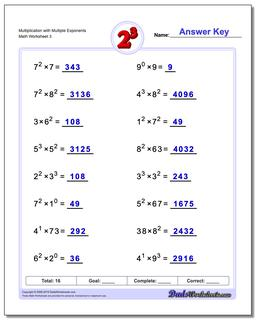# Math Worksheets: Exponents: Exponents: Multiplication with Multiple Exponents (Third Worksheet)## Multiplication with Multiple Exponents (Third Worksheet)

PropertyValue
DescriptionMultiplication with Multiple Exponents: Practice exponents worksheets mixing exponents with simple multiplication. Again, these are fantastic exercises for enhancing student comprehension of basic order of operations concepts where exponents are involved. (Third Worksheet)
Resource TypeWorksheet